# RD Sharma Solutions for Class 6 Maths Chapter 18: Basic Geometrical Tools Exercise 18.2

The major concept which is covered under this section is the method followed to measure the line segment using a ruler and divider. The solutions are prepared with pictorial representation to make it easy for the students during exam preparation. The solutions PDF can be downloaded by the students and used as a vital resource to perform well in the exam. The students who wish to score well in the exam can refer RD Sharma Solutions Class 6 Maths Chapter 18 Basic Geometrical Tools Exercise 18.2 PDF which are available here.

## RD Sharma Solutions for Class 6 Maths Chapter 18: Basic Geometrical Tools Exercise 18.2 Download PDF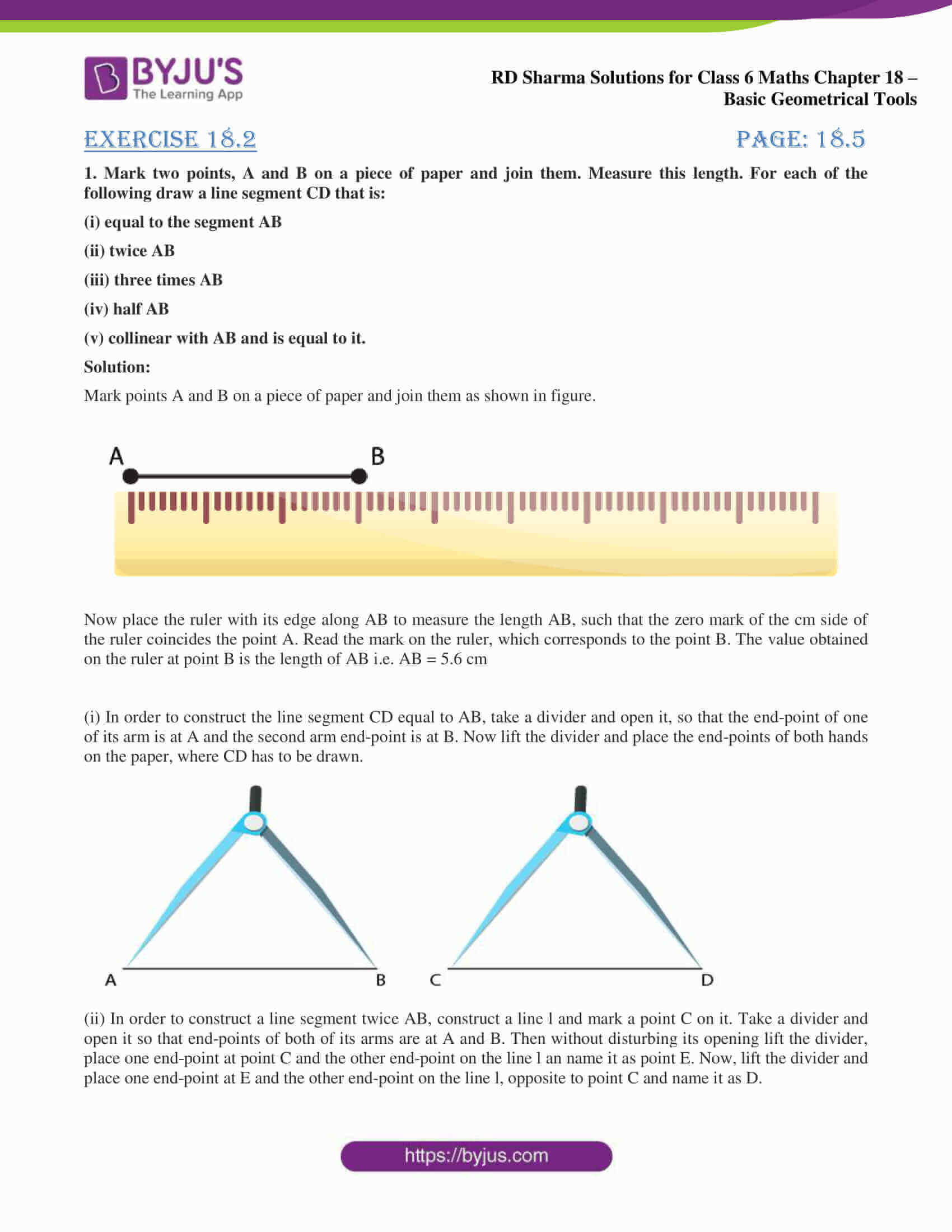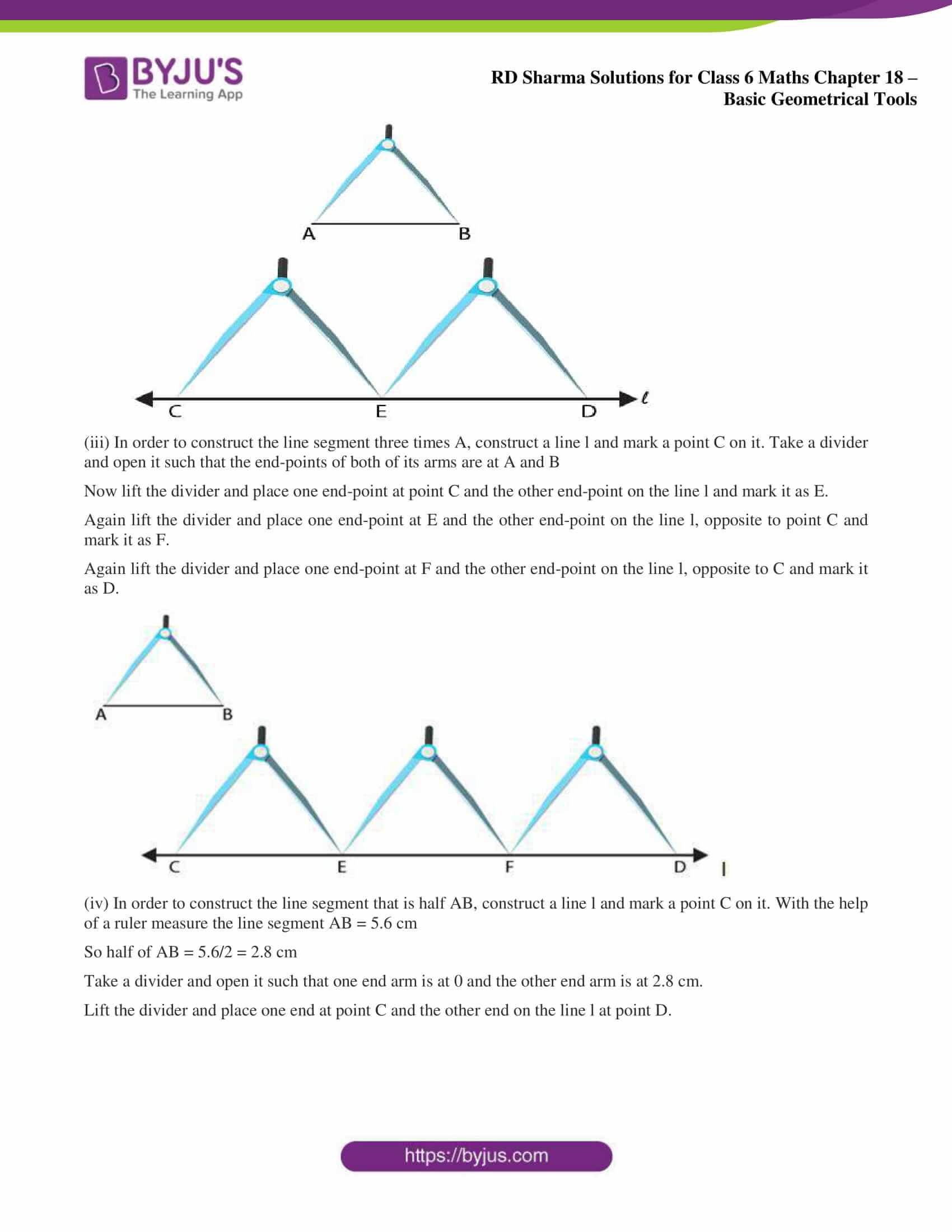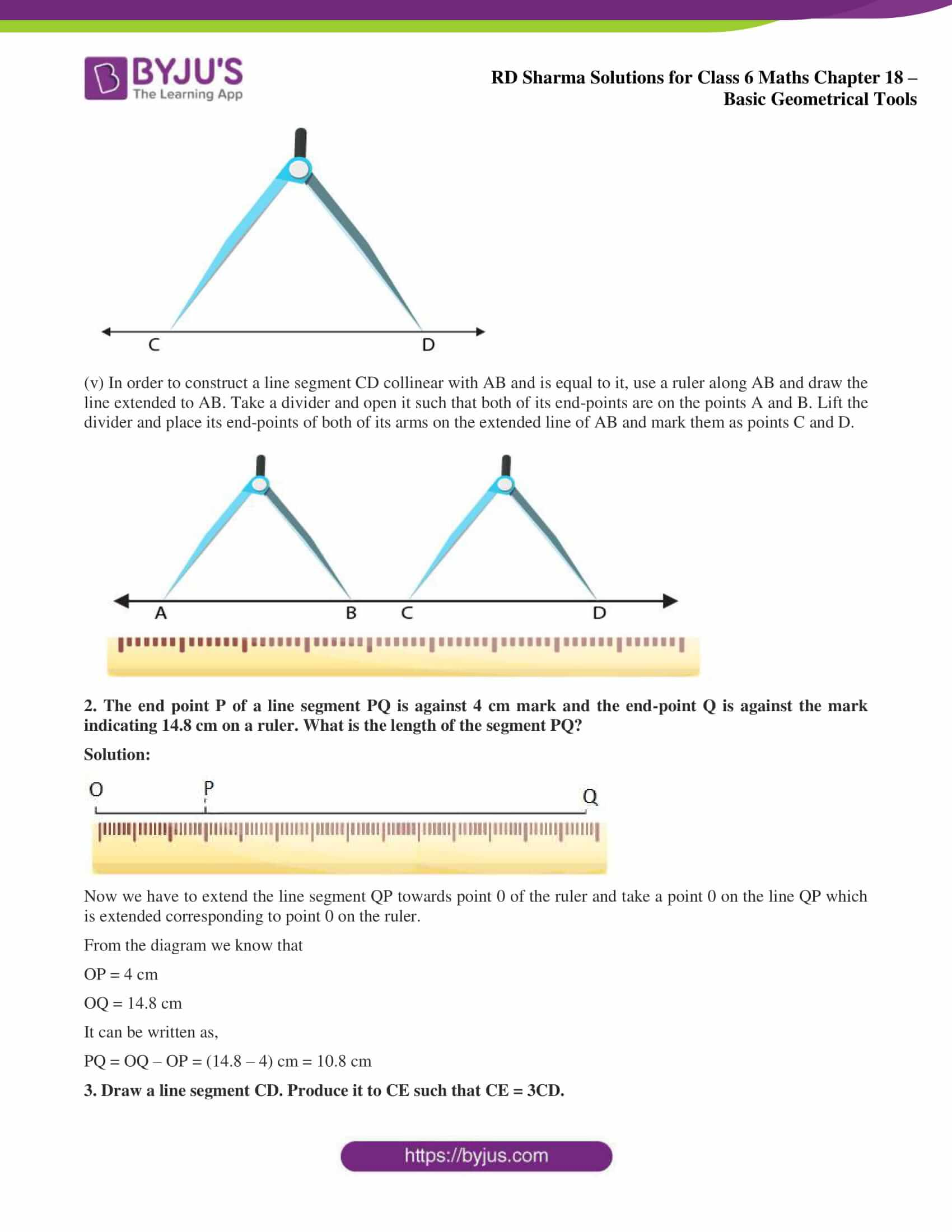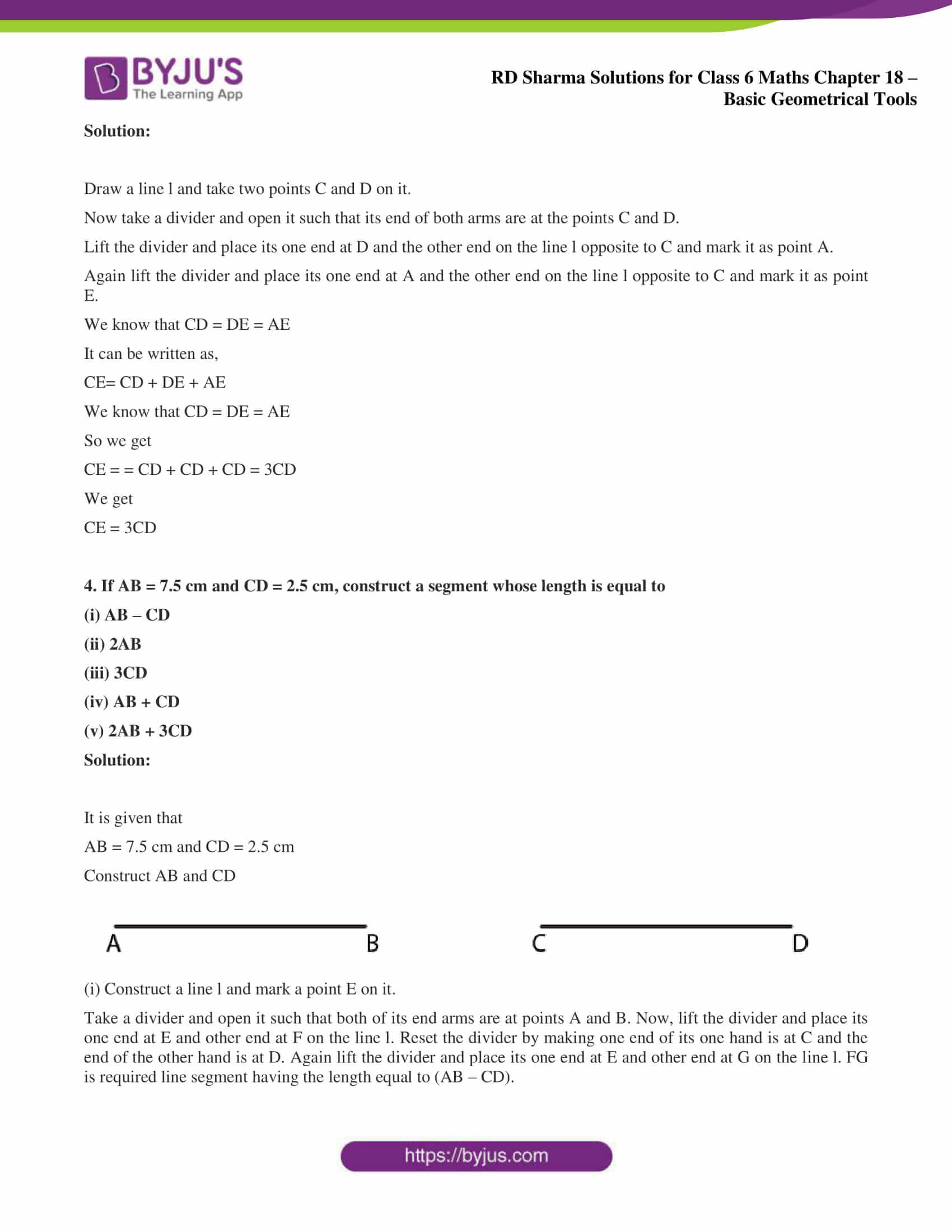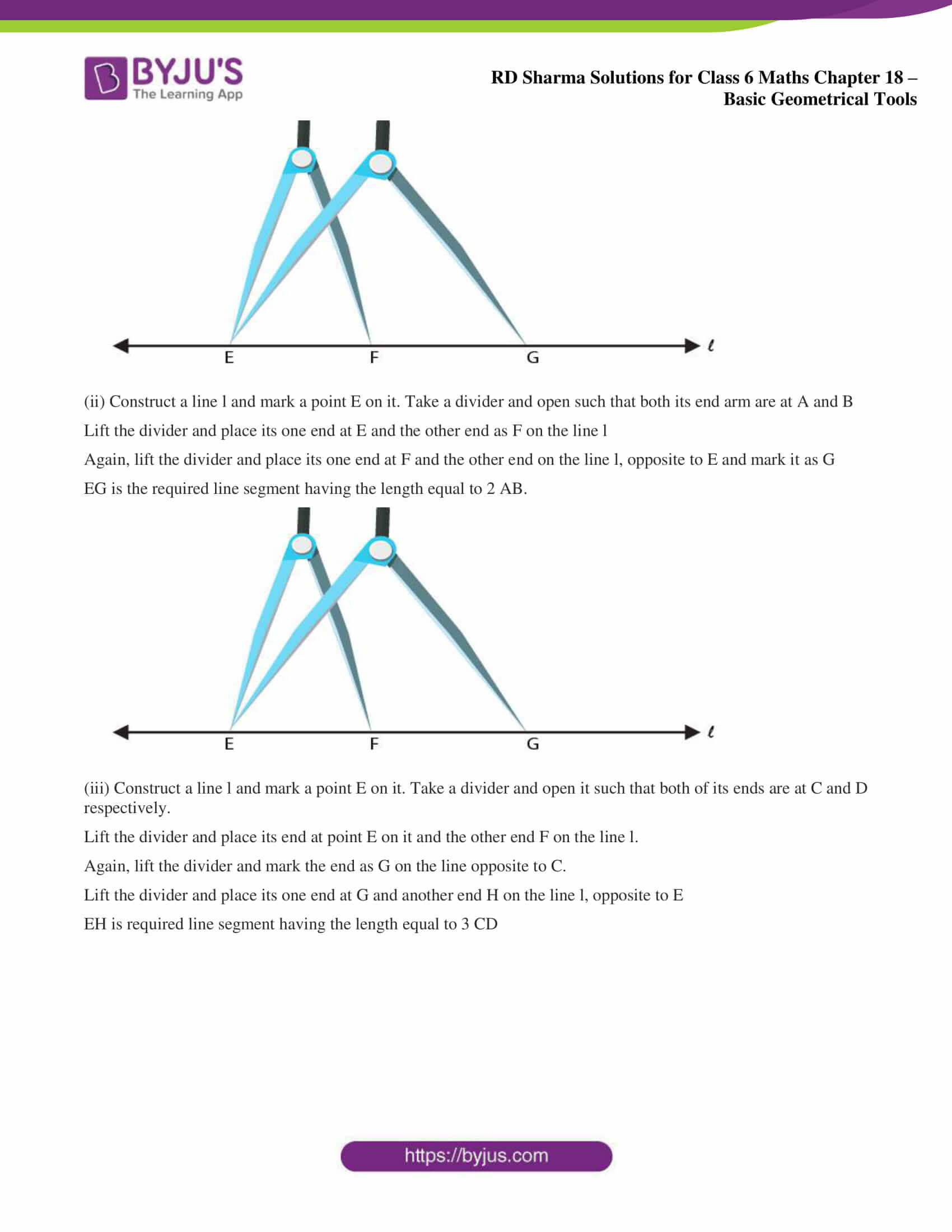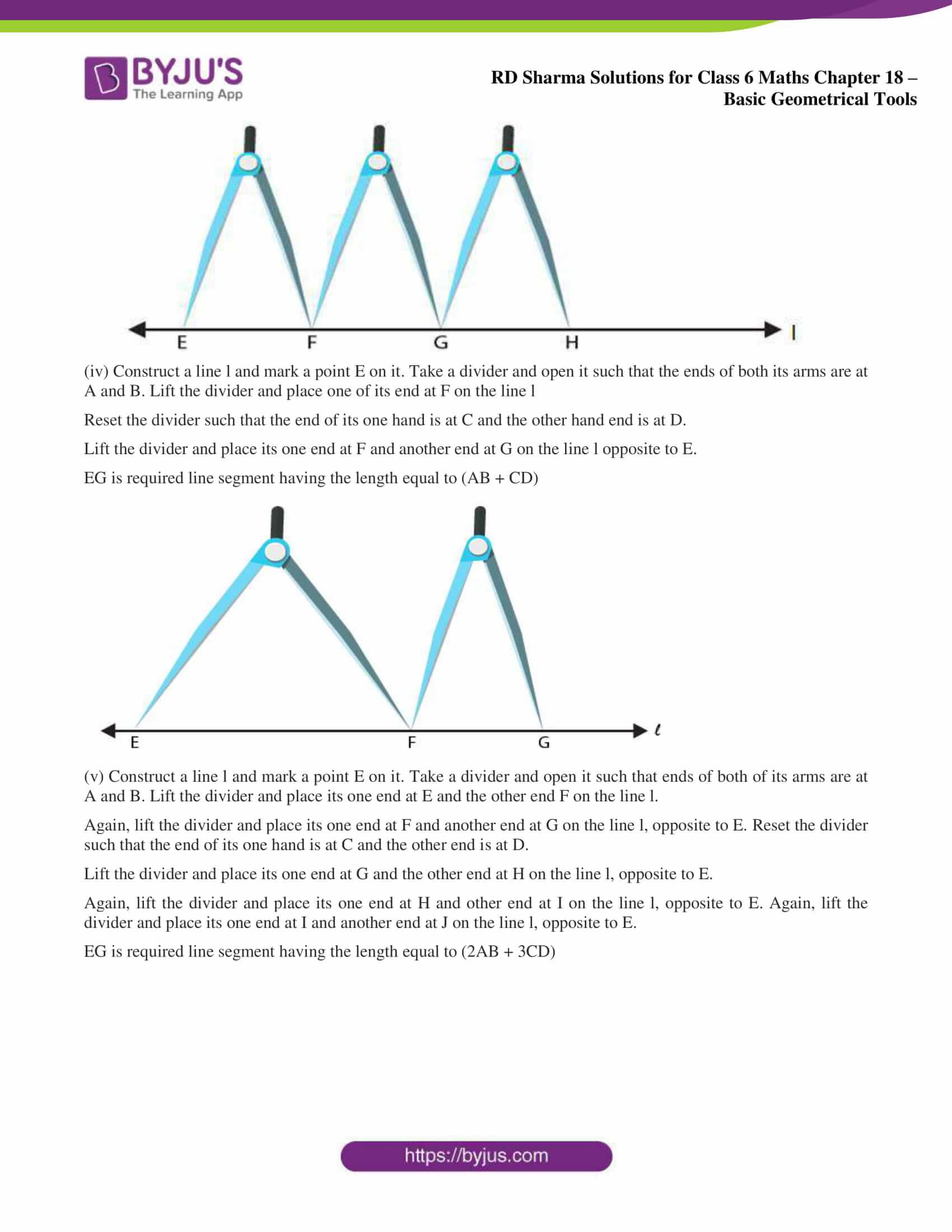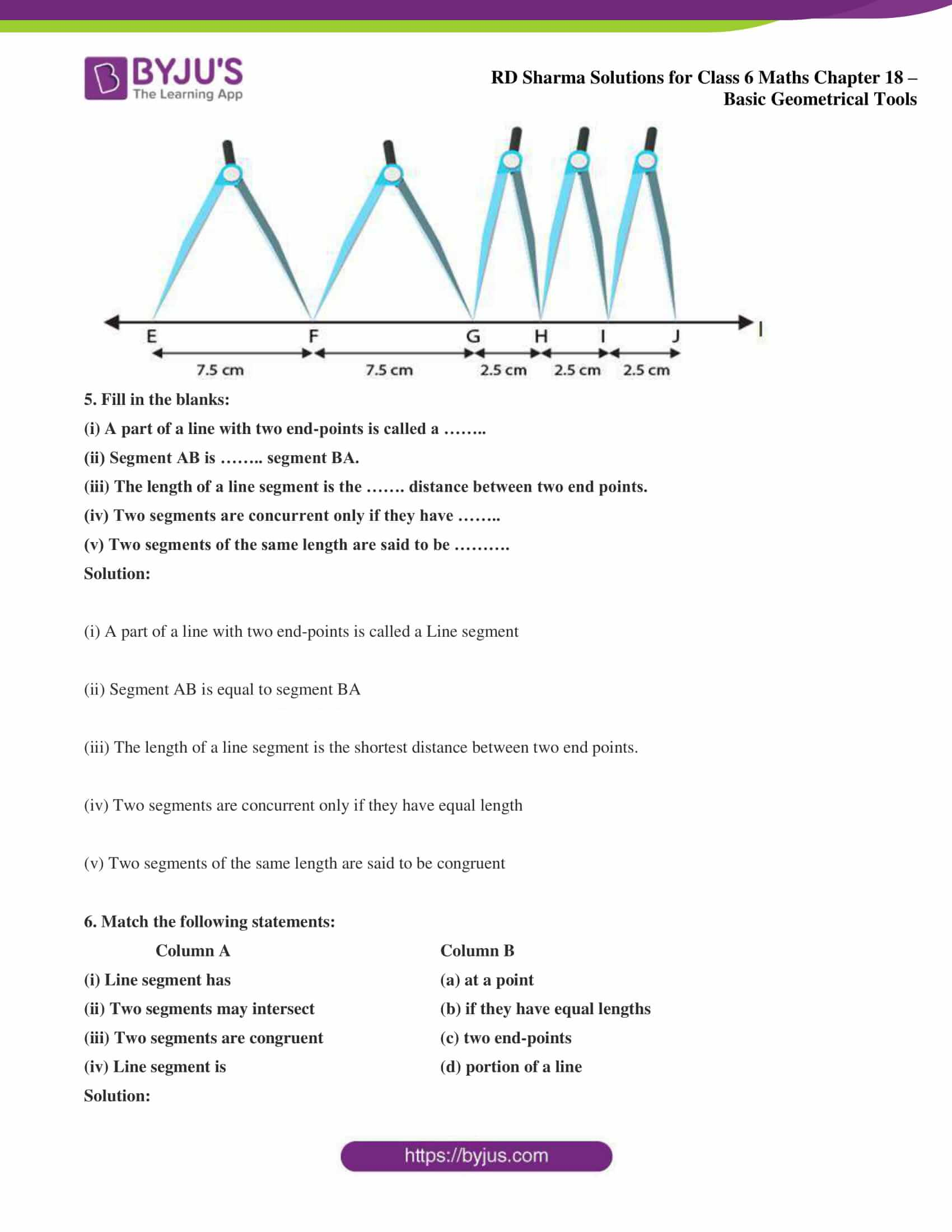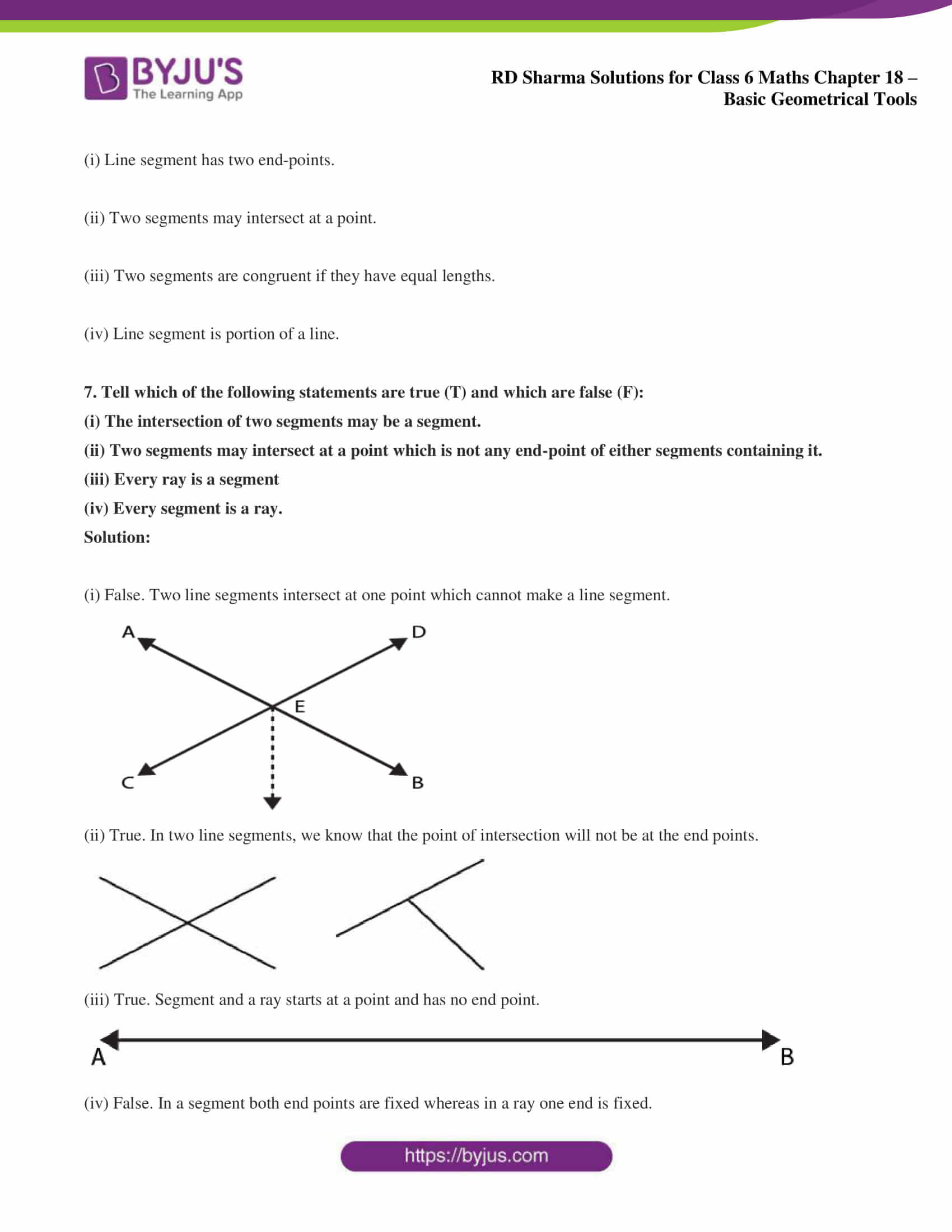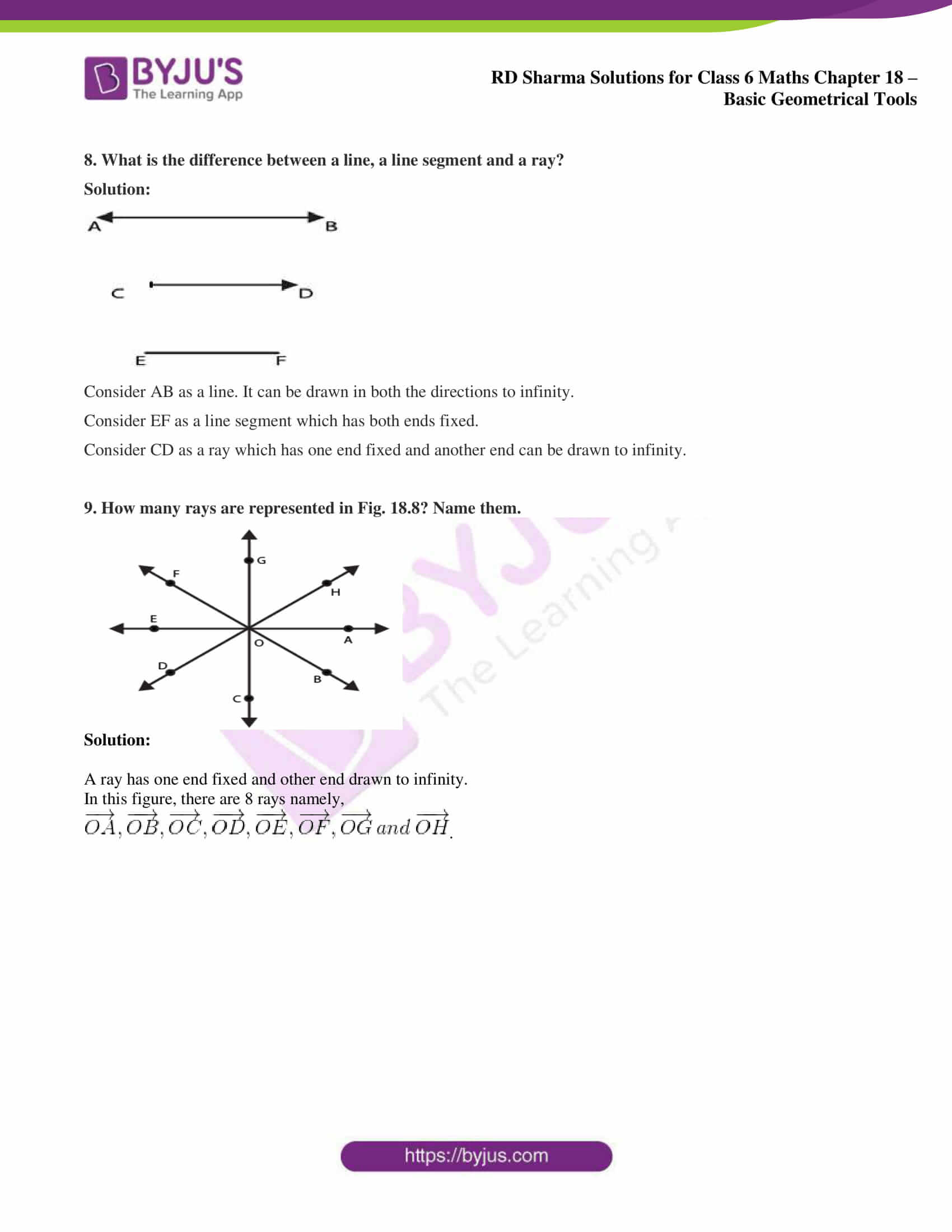### Exercise 18.2 page: 18.5

1. Mark two points, A and B on a piece of paper and join them. Measure this length. For each of the following draw a line segment CD that is:

(i) equal to the segment AB

(ii) twice AB

(iii) three times AB

(iv) half AB

(v) collinear with AB and is equal to it.

Solution:

Mark points A and B on a piece of paper and join them as shown in figure.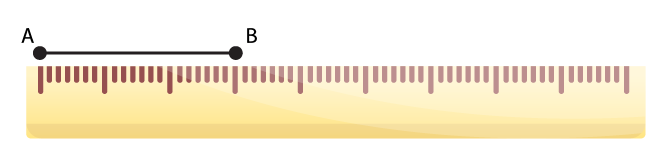Now place the ruler with its edge along AB to measure the length AB, such that the zero mark of the cm side of the ruler coincides the point A. Read the mark on the ruler, which corresponds to the point B. The value obtained on the ruler at point B is the length of AB i.e. AB = 5.6 cm

(i) In order to construct the line segment CD equal to AB, take a divider and open it, so that the end-point of one of its arm is at A and the second arm end-point is at B. Now lift the divider and place the end-points of both hands on the paper, where CD has to be drawn.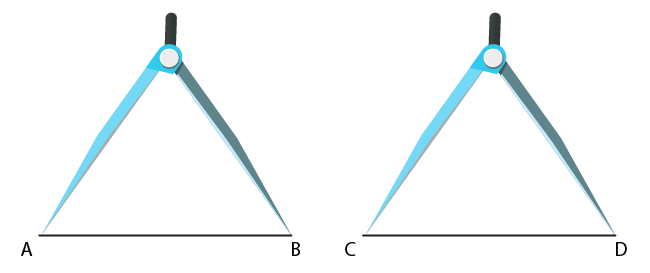(ii) In order to construct a line segment twice AB, construct a line l and mark a point C on it. Take a divider and open it so that end-points of both of its arms are at A and B. Then without disturbing its opening lift the divider, place one end-point at point C and the other end-point on the line l an name it as point E. Now, lift the divider and place one end-point at E and the other end-point on the line l, opposite to point C and name it as D.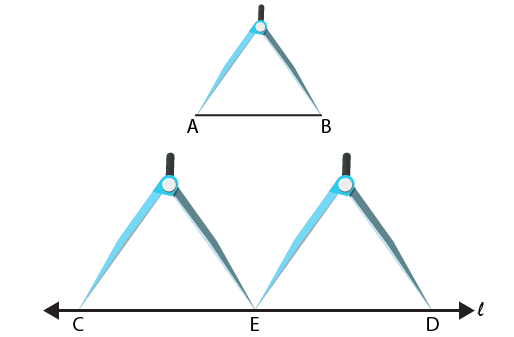(iii) In order to construct the line segment three times A, construct a line l and mark a point C on it. Take a divider and open it such that the end-points of both of its arms are at A and B

Now lift the divider and place one end-point at point C and the other end-point on the line l and mark it as E.

Again lift the divider and place one end-point at E and the other end-point on the line l, opposite to point C and mark it as F.

Again lift the divider and place one end-point at F and the other end-point on the line l, opposite to C and mark it as D.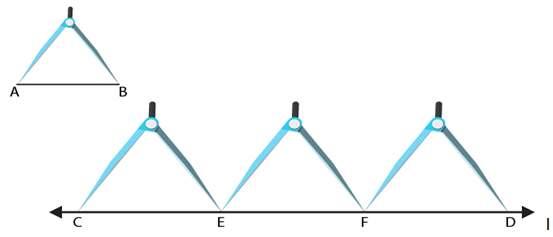(iv) In order to construct the line segment that is half AB, construct a line l and mark a point C on it. With the help of a ruler measure the line segment AB = 5.6 cm

So half of AB = 5.6/2 = 2.8 cm

Take a divider and open it such that one end arm is at 0 and the other end arm is at 2.8 cm.

Lift the divider and place one end at point C and the other end on the line l at point D.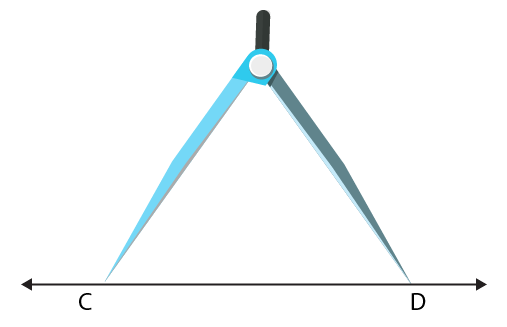(v) In order to construct a line segment CD collinear with AB and is equal to it, use a ruler along AB and draw the line extended to AB. Take a divider and open it such that both of its end-points are on the points A and B. Lift the divider and place its end-points of both of its arms on the extended line of AB and mark them as points C and D.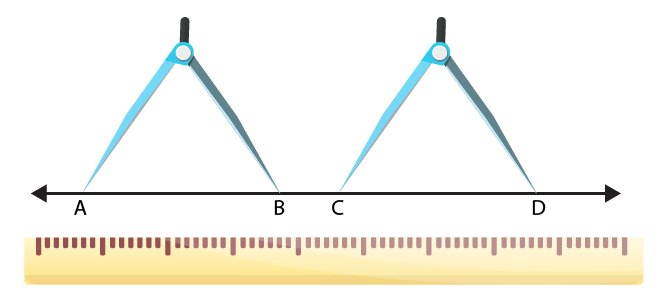2. The end point P of a line segment PQ is against 4 cm mark and the end-point Q is against the mark indicating 14.8 cm on a ruler. What is the length of the segment PQ?

Solution: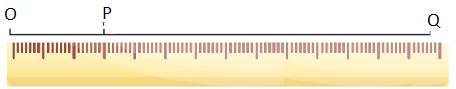Now we have to extend the line segment QP towards point 0 of the ruler and take a point 0 on the line QP which is extended corresponding to point 0 on the ruler.

From the diagram we know that

OP = 4 cm

OQ = 14.8 cm

It can be written as,

PQ = OQ – OP = (14.8 – 4) cm = 10.8 cm

3. Draw a line segment CD. Produce it to CE such that CE = 3CD.

Solution:

Draw a line l and take two points C and D on it.

Now take a divider and open it such that its end of both arms are at the points C and D.

Lift the divider and place its one end at D and the other end on the line l opposite to C and mark it as point A.

Again lift the divider and place its one end at A and the other end on the line l opposite to C and mark it as point E.

We know that CD = DE = AE

It can be written as,

CE= CD + DE + AE

We know that CD = DE = AE

So we get

CE = = CD + CD + CD = 3CD

We get

CE = 3CD

4. If AB = 7.5 cm and CD = 2.5 cm, construct a segment whose length is equal to

(i) AB – CD

(ii) 2AB

(iii) 3CD

(iv) AB + CD

(v) 2AB + 3CD

Solution:

It is given that

AB = 7.5 cm and CD = 2.5 cm

Construct AB and CD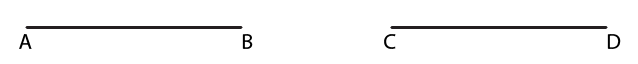(i) Construct a line l and mark a point E on it.

Take a divider and open it such that both of its end arms are at points A and B. Now, lift the divider and place its one end at E and other end at F on the line l. Reset the divider by making one end of its one hand is at C and the end of the other hand is at D. Again lift the divider and place its one end at E and other end at G on the line l. FG is required line segment having the length equal to (AB – CD).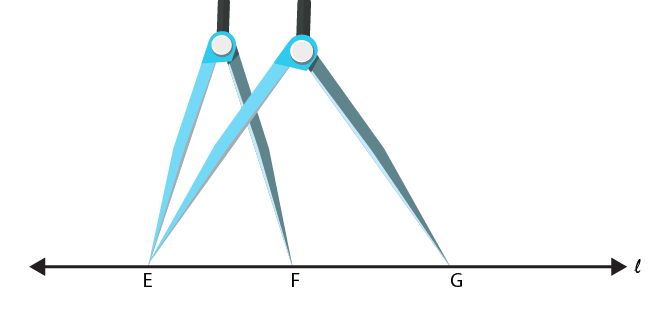(ii) Construct a line l and mark a point E on it. Take a divider and open such that both its end arm are at A and B

Lift the divider and place its one end at E and the other end as F on the line l

Again, lift the divider and place its one end at F and the other end on the line l, opposite to E and mark it as G

EG is the required line segment having the length equal to 2 AB.(iii) Construct a line l and mark a point E on it. Take a divider and open it such that both of its ends are at C and D respectively.

Lift the divider and place its end at point E on it and the other end F on the line l.

Again, lift the divider and mark the end as G on the line opposite to C.

Lift the divider and place its one end at G and another end H on the line l, opposite to E

EH is required line segment having the length equal to 3 CD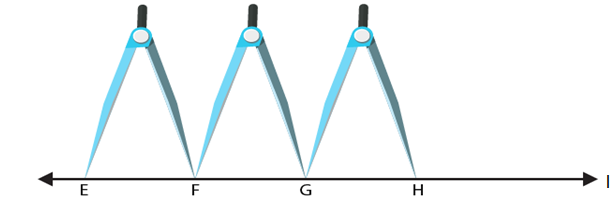(iv) Construct a line l and mark a point E on it. Take a divider and open it such that the ends of both its arms are at A and B. Lift the divider and place one of its end at F on the line l

Reset the divider such that the end of its one hand is at C and the other hand end is at D.

Lift the divider and place its one end at F and another end at G on the line l opposite to E.

EG is required line segment having the length equal to (AB + CD)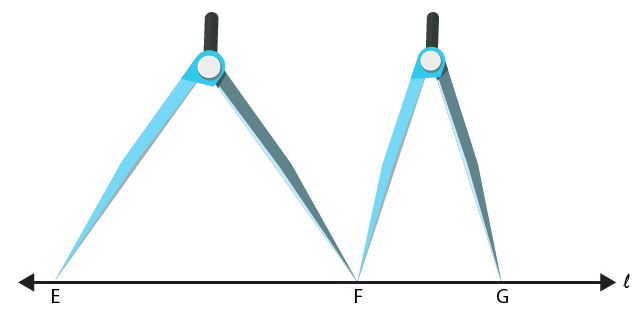(v) Construct a line l and mark a point E on it. Take a divider and open it such that ends of both of its arms are at A and B. Lift the divider and place its one end at E and the other end F on the line l.

Again, lift the divider and place its one end at F and another end at G on the line l, opposite to E. Reset the divider such that the end of its one hand is at C and the other end is at D.

Lift the divider and place its one end at G and the other end at H on the line l, opposite to E.

Again, lift the divider and place its one end at H and other end at I on the line l, opposite to E. Again, lift the divider and place its one end at I and another end at J on the line l, opposite to E.

EG is required line segment having the length equal to (2AB + 3CD)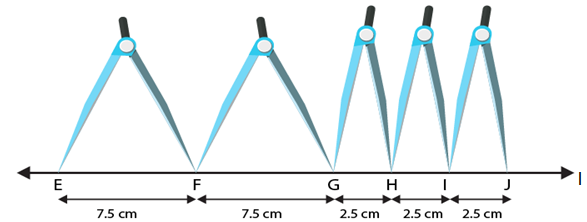5. Fill in the blanks:

(i) A part of a line with two end-points is called a ……..

(ii) Segment AB is …….. segment BA.

(iii) The length of a line segment is the ……. distance between two end points.

(iv) Two segments are concurrent only if they have ……..

(v) Two segments of the same length are said to be ……….

Solution:

(i) A part of a line with two end-points is called a Line segment

(ii) Segment AB is equal to segment BA

(iii) The length of a line segment is the shortest distance between two end points.

(iv) Two segments are concurrent only if they have equal length

(v) Two segments of the same length are said to be congruent

6. Match the following statements:

Column A Column B

(i) Line segment has (a) at a point

(ii) Two segments may intersect (b) if they have equal lengths

(iii) Two segments are congruent (c) two end-points

(iv) Line segment is (d) portion of a line

Solution:

(i) Line segment has two end-points.

(ii) Two segments may intersect at a point.

(iii) Two segments are congruent if they have equal lengths.

(iv) Line segment is portion of a line.

7. Tell which of the following statements are true (T) and which are false (F):

(i) The intersection of two segments may be a segment.

(ii) Two segments may intersect at a point which is not any end-point of either segments containing it.

(iii) Every ray is a segment

(iv) Every segment is a ray.

Solution:

(i) False. Two line segments intersect at one point which cannot make a line segment.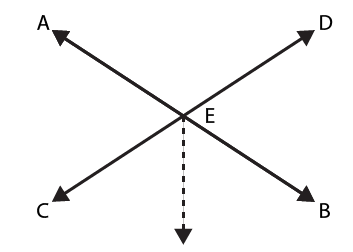(ii) True. In two line segments, we know that the point of intersection will not be at the end points.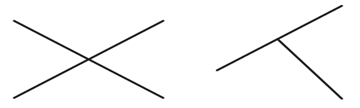(iii) True. Segment and a ray starts at a point and has no end point.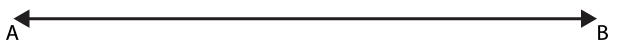(iv) False. In a segment both end points are fixed whereas in a ray one end is fixed.

8. What is the difference between a line, a line segment and a ray?

Solution: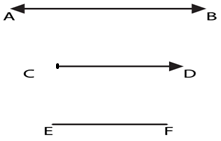Consider AB as a line. It can be drawn in both the directions to infinity.

Consider EF as a line segment which has both ends fixed.

Consider CD as a ray which has one end fixed and another end can be drawn to infinity.

9. How many rays are represented in Fig. 18.8? Name them.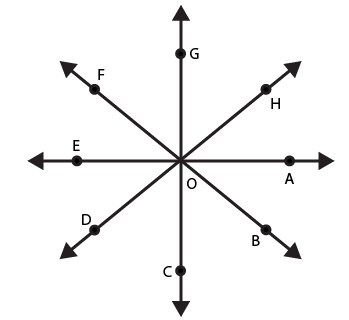Solution:

A ray has one end fixed and other end drawn to infinity.

In this figure, there are 8 rays namely,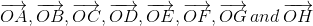.